# Character Data Types in C

## Character Data Types in C Language with Examples

In this article, I am going to discuss Character Data Types in C Language with Examples. Please read our previous article where we discussed Integer Data Types in C Language. At the end of this article, you will understand everything about character data type in c language.

##### Character Data Types in C Language

The character data type is divided into two types one is signed data type and the second one is unsigned data type.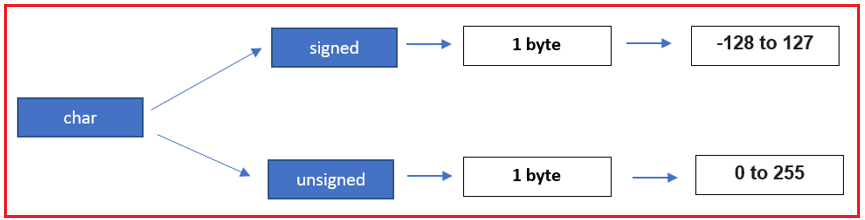Both Signed data type and unsigned data type occupy only one byte of memory. Unsigned means it will accept only positive values and the signed means it will accept both positive as well as negative values. Whatever the type either signed or unsigned, the character occupies only one byte.

##### Using 1 byte of memory what is the minimum and maximum value we can store?

To understand this, look at the memory allocation process. Here I am taking 1 byte of memory. 1 byte equals 8 bits. And it takes only binary values i.e. 0 and 1. Now, if we place zeros in all 8 places then the value will be zero which is the minimum we can store in a 1-byte memory location as shown in the below image.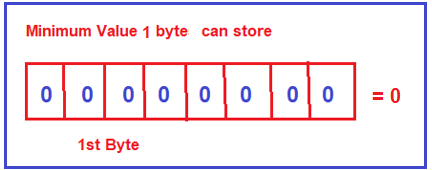If we place all ones in all the 8 bits, the value is 255. So, the maximum integer value we can store in 1 byte is 255 as shown in the below image.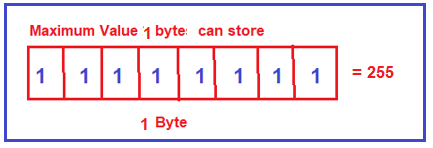So, using 1 byte of memory, the minimum integer value we can store is 0 and the maximum integer value we can store is 255.

##### Unsigned Character Range in C Language:

As we already discussed unsigned means it will accept only positive values. And the range 28 equals 256. As positive value starts with 0, so, the unsigned character data type range is from 0 to 255.

##### Signed Character Range in C Language:

Now let us understand the range of Signed character data types. The signed data type accepts both positive as well as negative values. So, we need to divide 28 = 256 by 2. 256/2 the value is 128. So negative values start with -1, -2, and up to -128 and the positive values start from 0 up to 127.

We are using character data type to store symbols like a, b, A, B, or some special symbols. Then how can we represent such symbols in integers? Why character data type representation in integer. So, while working with Character Data Types in C Language, we need to understand the following four questions.

1. Why does character limits representation in integers?
2. How can we store symbols into one-byte memory nothing but why character occupies one-byte memory?
3. What is a character system?
4. What is ASCII?

Consider the below diagram. It is a simple program and we name this program as Program.c, and inside the main method, we are declaring one integer local variable and assigned with a value of 10 and the remaining instructions are also there as it is. We can call it source code.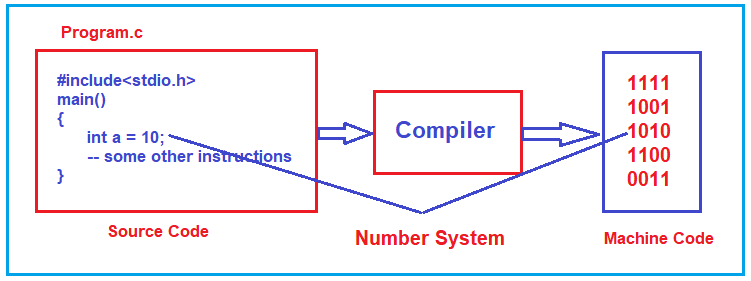In our previous article, we already discussed that whatever program we have written using any high-level programming language that the system cannot understand. This is because the system can understand only binary language. But you have written an English statement. We should convert all these high-level instructions into low-level. Who will convert? The Answer is the compiler.

The compiler is a predefined program. We need to pass the source code to the compiler and the compiler will then generate the binary instructions code which is in the form of zeros and ones. So, the compiler needs to convert all these high-level instructions into machine level. Consider 10, it will convert into binary i.e. 1010 and this is possible by using the number system. So, using the number system concept we can convert the decimal value to binary value.

But here the problem is how it is converted #, <, >, a, I, A, etc. symbols into binary. If the decimal value is there, we can use a number system to convert it into binary. But how we can convert characters (a, b, A, B) and special symbols (#, <. >, etc.) into binary? The answer is the character system. Only for computer programming languages, the character system was introduced.

##### What is a character system?

Using a character system, we can represent one entire language into integer constants. For example, the English language contains capital letters, small letters, digits special symbols, etc., and using a character system we can represent all the above characters and symbols into integer constants. This is called a character system.

##### How many character systems are available?

A list will come if you search on google. A number of character systems are available. The first computer was introduced into the market by IBM. IBM is having its own character system. Now, the famous one is the ASCII character system, and every programming language follows the ASCII character system only. Let us see how using the ASCII character system, we can represent one particular language.

##### What is ASCII? What it stands for?

Now let us understand the English language ASCII Code. ASCII stands for Americans Standard Code for Information Interchange. A standard code means it is a fixed code, no one can change the value and no one can modify the value. It is used to interchange the information from high-level language to low-level language.

##### How ASCII represents?

To understand how ASCII represents the English language, please have a look at the below diagram,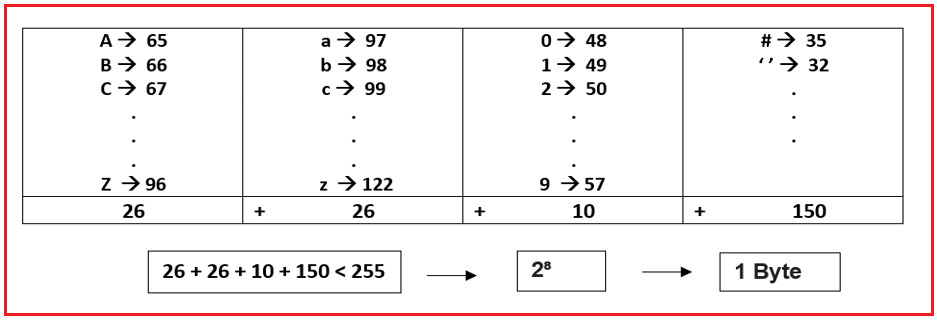As you can see in the above image, capital A is represented by a constant integer value 65 and this is the fixed value and no one can change this value. The next one is for capital B and is 66 and for capital C it is 67, so on and for capital Z it is 90. The value of small a is 97 and small B is 98 and so on up to small z whose value is 122.

For digit 0, the ASCII value is 48, for 1 the value is 49 and for 9, the ASCII value is 57. Using the digits 0 to 1, you can construct any number, so they have given ASCII for 0 to 9 only.

For special characters, if it is a space the value is 32, for #, the value is 35, and so on for every symbol. So, every character, digit, special symbol, is represented by a constant integer value in the character system. Not only in the ASCII character system but in any character system which is available in the market.

So, for every language like English, Hindi, Odia, there is a character system. Here, the above diagram representing the English language using the ASCII character system, and these are the standard values.

##### How can we store a symbol into one-byte memory?Just count all the values, so total will 26 capital alphabets we have in English and 26 small letters and next 10 numbers and not more than 150 special symbols. So here if you add all these, then this is less than 256. Any language you can take in this world, it is at most having 256 symbols. So, ASCII decided that if we assign the values for these symbols from 0 to 255, so you can represent any character in the language using one byte of memory.

##### How can we say that one-byte memory?

256 is nothing but a 2 power 8 value. 2 power 8 is nothing but a one-byte memory. This is the only reason every character we can represent using one byte of memory in a programming language.

##### Character Data Types Examples in C Language

Now we will see some of the examples on the character data type. First, let us understand the unsigned character and signed character in the form of circles.

##### Understanding signed char data type circle in c Language.

If it is a signed character the limits are from -128 to +127. Let us write all these limits in the form of a circle and based on these circles only we will see how programs will execute.

Either positive value or negative value always the counting starts with 0. Positive value counting starts from 0, 1, 2, and so on up to 127 in a clockwise direction, and here the maximum positive value is 127. Negative values counting starts from -1, -2, -3, and so in up to -128 in an anti-clockwise direction as shown in the below image.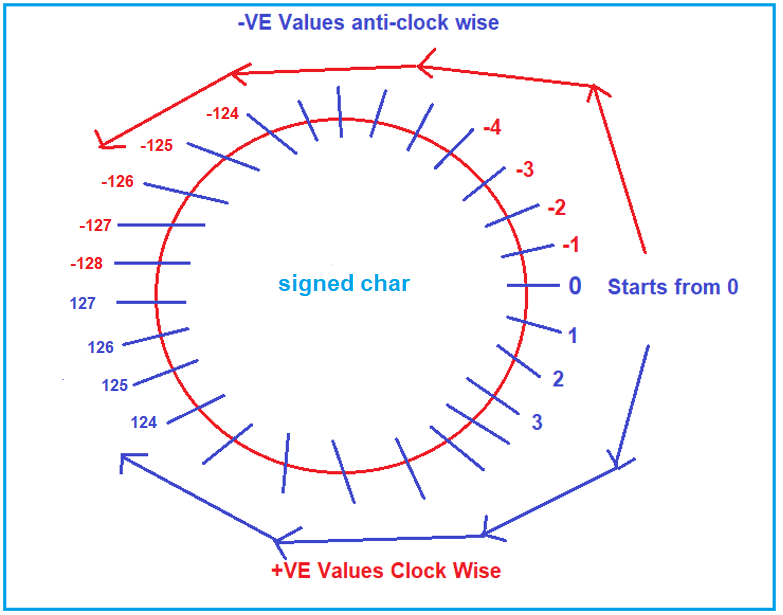Note: In the declaration of variable if you are not specifying whether the variable is a signed variable or unsigned variable by default it is a signed variable and can accept both positive and negative values.

##### Understanding unsigned char data type circle in c Language.

If it is an unsigned character the limits are from 0 to 255 and the unsigned char data type accepts only positive values. In the case of unsigned char, the circle starts from 0, 1, 2, so on and ends with 255 i.e. the maximum positive value is 255 as shown in the below image.

#####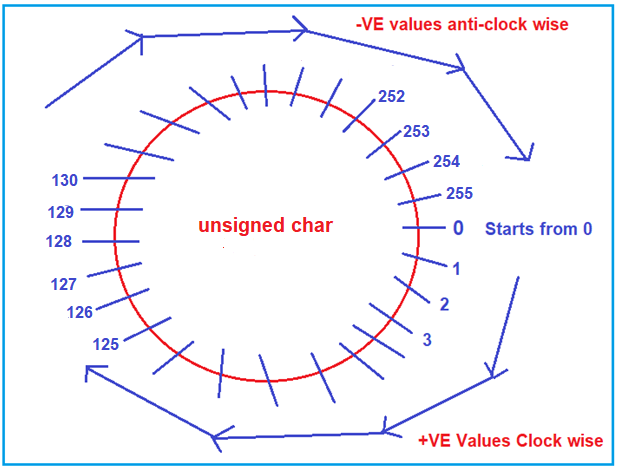Example to understand character data type in c language:

Following is a simple example of a c program using char data type. Here, inside the main function, we are declaring one character variable with the name CH (you can give any name as per your choice) and assigning this variable with the value A. In the C programming language, we are representing characters by using single quotes. Then we are printing the character in the console. To print the character in the console we need to use the format specifier as %c. %c is the format specifier for character and it will print the value A in the console. Next, we have also written the %d format specifier for the character variable CH. In this case what it will print? Here, it will print the corresponding ASCII value of character A which is nothing but 65.

```#include <stdio.h>
int main()
{
char CH = 'A';
printf("%c", CH);
printf(" %d", CH);
return 0;
}
```

Output: A 65

Now we will see some tricky questions on character data type which are mostly asked in interviews.

```#include <stdio.h>
int main()
{
char CH = 258;
printf("%c", CH);
printf(" %d", CH);
return 0;
}
```

In the above example, we have initialized the character variable CH with a value of 258. Yes, we can store integers on the character data type. The above CH variable is by default a signed character. So, to understand what value it will store we need to understand the signed char circle and see the actual value of 258. As 258 is a positive value, so the count will start from 0, 1, and so on in a clockwise direction. In the circle when it reached 127, the next value is -128 (in count it will be 128), the next is -127 (in count it will be 129), and in the same way, -1 will be for 255, the next value in the circle is 0 which is 256, 1 for 257, and 2 for 258. So, in the variable instead of 258, it will store.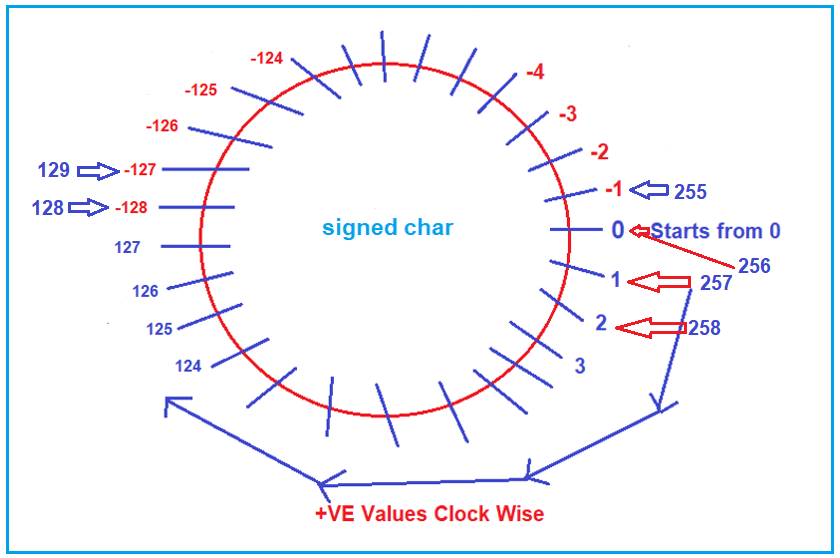So, in the output for %d format specifier, it will print 2 and for character specifier, it will print some unknown value i.e. 2 corresponding unknown characters it will print from the ASCII character system and when you run the above code, you will get the following output.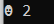Now, we will see how to write a program in which we will input one character and it has to print that corresponding ASCII value. In this program, we are going to work with the scanner function. Using the scanf function, we are taking input from the end-user in the C programming language.

###### What is a console?

The console is where you can see the output and where we can give the input.

##### Write a program to display ASCII value of character input by end-user.

We want to read information from the end-user while the application is executing is our concept. We are reading information from end-user i.e. we are taking input from the end-user. Please have a look at the below program. Here, I am declaring one character variable with the name CH i.e. char CH; here, the variable CH gets memory allocation.

On the console first, we print a message and asking the end-user to enter a character. We are reading the end-user input using the scanner function. The scanner function is available in stdio.h header file.

What end-user want to do they cannot understand that, so that is the reason we need to provide some information. Best example atm application, whenever you enter into an atm center clear information it will show please choose one language, insert your atm card, insert your pin number and how much amount you want to withdraw. Information is very important, how good you are writing the logic is not at all matters. First, we are asking the message very clearly i.e. enter one character. Whatever the message you have written in the printf function, that will be written on the console. It will print that message enter a character.

Whenever the end-user will enter one character, for example, the end-user entered a character h. Then automatically system will print the ASCII value of h. h should be stored in some memory location. So here we need to provide a memory address. How to provide a memory address, who will provide a memory address? So, for the first time, we are using scanf function in C programming.

If you want to read only one character, so write one time %c format specifier. If you want to read ten characters then you have to write %c 10 times. But here it is only one character, so here we are specifying the character address by using the & address operator. It will return the address of the memory location of the CH variable. Whatever character we have given in the console will be stored in that location. h corresponding ASCII value will be converted into binary and then the binary value will go and store in that memory location. Now we want to print the ASCII value just printf “ASCII Value is %d” and output will be generated. Whatever we discussed above is given in the below example.

```#include <stdio.h>
int main()
{
char CH;
printf("Enter a Character : ");
scanf("%c", &CH);
printf("ASCII Value is %d", CH);
return 0;
}
```
###### Output: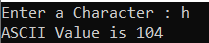In the next article, I am going to discuss Sizeof() function and limits.h header file in C language with Examples. Here, in this article, I try to explain Character Data Types in C Language with Examples and I hope you enjoy this Character Data Types in C Language with Example article.